Next: 1.6 Russell's Paradox Up: 1. Notation, Undefined Concepts Previous: 1.4 More Sets   Index

# 1.5 Functions

1.57   Definition (Function.) Let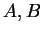be sets, and let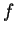be a rule that assigns to each elementin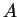a unique element (denoted by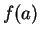) in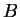. The ordered triple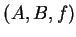is called a function with domainand codomain. We write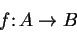to indicate thatis a function. It follows from the definition that two functions are equal if and only if they have the same domain, the same codomain, and the same rule: If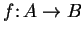and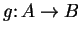, I say that the ruleand the ruleare the same if and only iffor all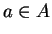. We usually say  the function" when we mean  the function," i.e., we name a function by giving just the name for its rule.

1.58   Examples. Let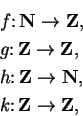be defined by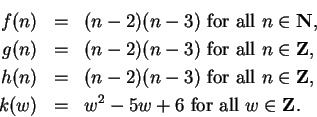Then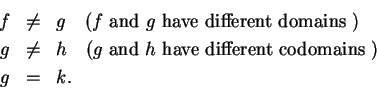Ifis a proposition form on a set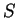, thendetermines a function whose domain isand whose codomain is the set of all propositions.Next: 1.6 Russell's Paradox Up: 1. Notation, Undefined Concepts Previous: 1.4 More Sets   Index